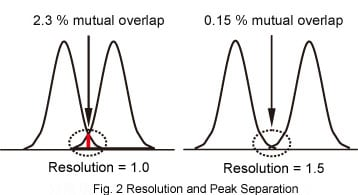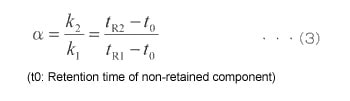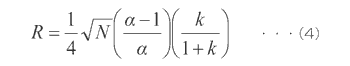### What is Multidimensional Chromatography?

Multidimensional chromatography uses a combination of several chromatography techniques, separation modes, and columns to separate multiple components. It achieves significantly higher separation than normal one-dimensional chromatography. Various separation modes and the corresponding mobile phases can be selected for HPLC, and the diverse permutations available suggest the possibility of achieving a degree of selectivity not possible using one-dimensional separation alone.

Equation (1) indicates that the resolution is the difference between peak retention times divided by the average peak width. In a peak with Gaussian distribution, the peak width is W = 4 σ (where σ is the standard deviation) and the peak FWHM is W0.5h = 2.354σ. Substituting these relationships into equation (1) gives results in equation (2).

### Resolution and Peak Separation

The resolution is represented as a numeric value, such as 0.8, 1.0, or 3.0. But what is the relationship between the number representing the resolution and the actual peak separation? At a resolution of 1.0, if the two peaks are assumed to have a Gaussian distribution and have the same peak height and peak width, then the difference in retention time from equation (1) becomes 1.0W, or 1.0 × 4σ = 4 σ. In the case of a Gaussian distribution, 4 σ encompasses 95.4 %, such that the peaks overlap by 2.3 % ((100 % - 95.4 %)/2). This indicates that 2.3 % of the peak intrudes into the other peak from a perpendicular line drawn in the trough. Similarly, a resolution of 1.5 indicates a difference in retention time of 1.5× 4σ = 6σ, which corresponds to an overlap of 0.15 % ((100 % - 99.7 %)/2). See Fig. 2.### Resolution and Separation Factor, Retention Factor, Number of Theoretical Plates

In addition to resolution, the separation factor (α) is also used as an indicator of the separation of two peaks. The separation factor is defined as the ratio of the retention factors (k), as shown in equation (3).The resolution can be expressed in terms of the number of theoretical plates, separation factor, and retention factor, as shown in equation (4).